#Systems with Zero as an EigenvalueWe discussed the case of system with two distinct real eigenvalues, repeated (nonzero) eigenvalue, and complex eigenvalues. But we did not discuss the case when one of the eigenvalues is zero. In fact, it is easy to see that this happen if and only if we have more than one equilibrium point (which is (0,0)). In this case, we will have a line of equilibrium points (the direction vector for this line is the eigenvector associated to the eigenvalue zero).

Example. Find the general solution to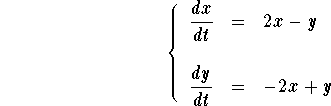Answer. The characteristic polynomial of this system is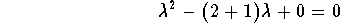which reduces to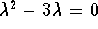. The eigenvalues are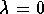and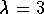. Let us find the associated eigenvectors.For, set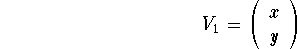The equation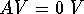translates into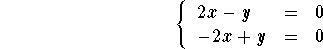The two equations are the same. So we have y = 2x. Hence an eigenvector is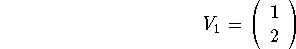For, set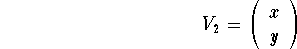The equation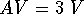translates into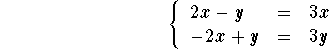The two equations are the same (as -x-y=0). So we have y = -x. Hence an eigenvector is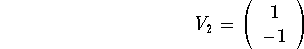Therefore the general solution is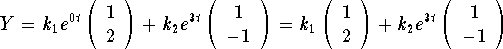Note that all the solutions are line parallel to the vector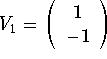. When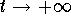, the trajectory goes to infinity. But when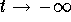, the trajectory converge to the equilibrium point on the line of equilibrium points (that is passing by (0,0) and having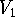as a direction vector). The picture below explains more what is happening.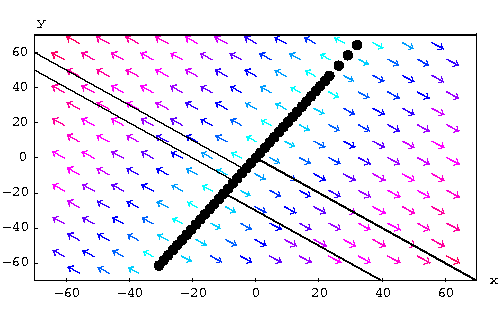The general case is very similar to this example. Indeed, assume that a system has 0 and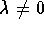as eigenvalues. Hence ifis an eigenvector associated to 0 and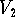an eigenvector associated to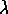, then the general solution is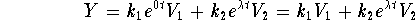We have two cases, whether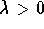or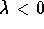.If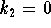, then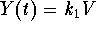is an equilibrium point.If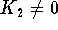, then the solution is a line parallel to the vector. Moreover, we have whenif, the solution tends away from the line of equilibrium;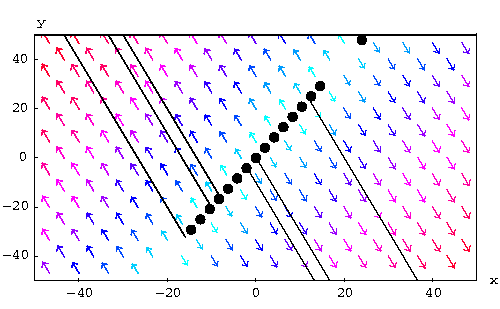if, the solution tends to the equilibrium point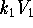along a line parallel to.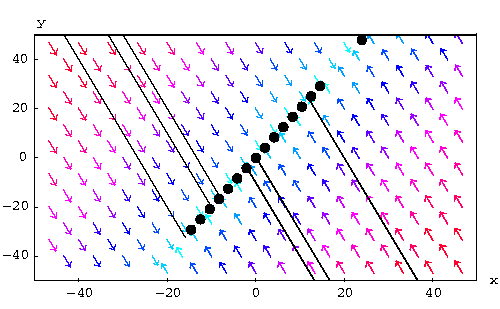[Differential Equations]
[Geometry] [Algebra] [Trigonometry ]
[Calculus] [Complex Variables] [Matrix Algebra]S.O.S MATHematics home page

Do you need more help? Please post your question on our S.O.S. Mathematics CyberBoard.Author: Mohamed Amine Khamsi